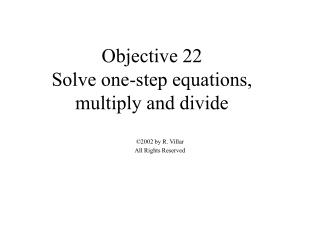DownloadDownload PresentationObjective 22 Solve one-step equations, multiply and divide

# Objective 22 Solve one-step equations, multiply and divide

Download Presentation## Objective 22 Solve one-step equations, multiply and divide

- - - - - - - - - - - - - - - - - - - - - - - - - - - E N D - - - - - - - - - - - - - - - - - - - - - - - - - - -